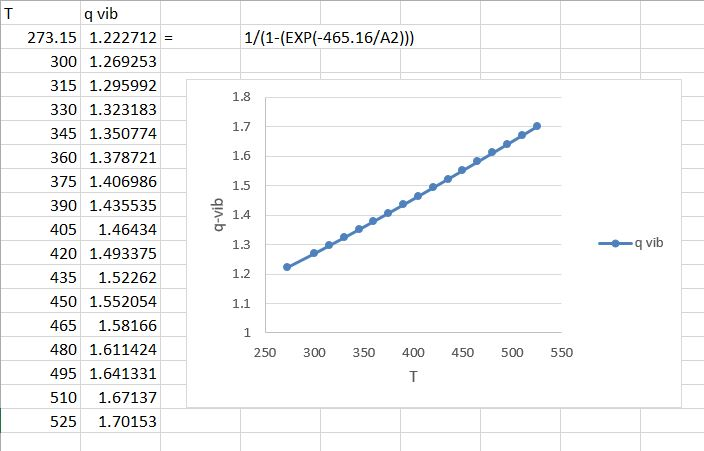# Question & Answer: The vibrational wavenumber of Br2 is 323.2 cm-1. a) Evaluate the vibrational partition function…..

The vibrational wavenumber of Br2 is 323.2 cm-1.
a) Evaluate the vibrational partition function
without approximation and plot its value as a function of temperature.
b) Determine the temperature at which the value obtained lies within 5% of the approximate value.

Don't use plagiarized sources. Get Your Custom Essay on
Question & Answer: The vibrational wavenumber of Br2 is 323.2 cm-1. a) Evaluate the vibrational partition function…..
GET AN ESSAY WRITTEN FOR YOU FROM AS LOW AS \$13/PAGE

A) The vibrational partition function for diatomic particles is given by:

qvib =

= 323.2 cm-1

h – planks constant

k – stefen boltzman constant

-h/k = = 465.16 K

Plot:

q vib = 1/(1-exp(465.16/T))

At room temp i.e 300 K

/T = 1.55B) At room temperatue 300K qvib = 1.26. This implies, as before, that very few vibrational states are accessible.

temperature at which the value obtained lies within +5% of the approximate value is 300 K to 330K